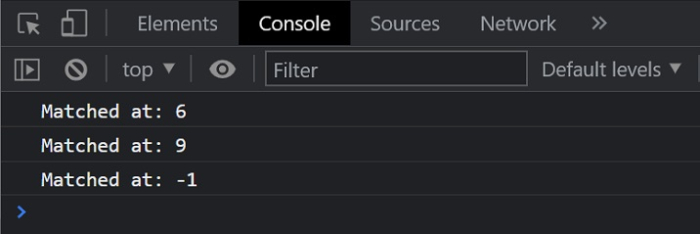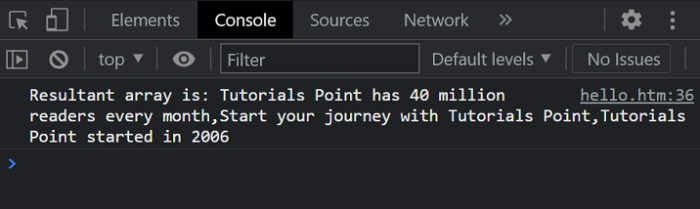# How to return all matching strings against a RegEx in JavaScript?

In this article, we will explore the essentials of a regular expression (RegEx) and how to compare other strings with this regex in JavaScript. We will learn how to identify a similar string with that of a regex and then subsequently return them. We can use the string.search() method to match the regular expression in a given string.

## Syntax

let index = string.search(expression)

## Parameters

• string − This is the string that will be searched in comparison with the expression.

• expression − This is the regular expression that will be used for checking with the original string.

## Example 1

In the below example, we are going to compare a string with a substring/regular expression that is rendered in the function. This method will only return the elements that match the expression, else the string will not be returned.

# index.html

<!DOCTYPE html>
<html>
<title>
Comparing Strings
</title>
<body>
<h2 style="color:green">
Welcome To Tutorials Point
</h2>
</body>
<script>
// Taking a String input.
var string = "Start Learning new technologies now.";

// Defining a regular expression
var regexp1 = /Lea/;
var regexp2 = /rn/;
var regexp3 = /abc/;
console.log("Matched at:"+string.search(regexp1));

// Expected Output: 6
console.log("Matched at:"+string.search(regexp2));

// Expected Output: 9
console.log("Matched at:"+string.search(regexp3));

// Expected Output: -1
</script>
</html>

## Output## Example 2

# index.html

<!DOCTYPE html>
<html>
<title>
Comparing Strings
</title>
<body>
<h2 style="color:green">
Welcome To Tutorials Point
</h2>
</body>
<script>
// Taking input an array of strings.
let input = [
"Tutorials Point has 40 million readers every month",
"Start your journey with Tutorials Point",
"It started in 2006",
"Start Learning",
"Tutorials Point started in 2006"
];
let i;
// Defining the regular expression
let regexp = /Tutorials/;
let result = [];
for (i = 0; i < input.length; i++) {
if (input[i].search(regexp) != -1) {
result.push(input[i])
}
}
console.log("Resultant array is:"+result)
</script>
</html>

## Output